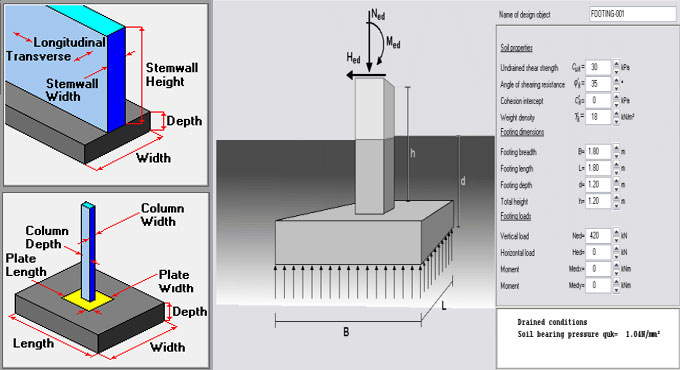# Some useful guidelines to work out the total loads on a column & footingThe following types of loads operate on a column :-

1. Self weight of the column x Number of floors
2. Self weight of beams per running meter
3. Load of walls per running meter

The columns are also susceptible to bending moments which should be included in creating the final design. There are different types of advanced structural design software like ETABS or STAAD Pro which can be applied to design a good structure efficiently. The calculation for structural loading In professional practice is based on some fundamental assumptions.

For Columns: Self weight of Concrete is approximately 2400 kg per cubic meter that is identical to 240 kN. Self weight of Steel is approximately 8000 kg per cubic meter. Suppose a large column having size of 230 mm x 600 mm with 1% steel and 3 meters standard height, the self weight of column is approximately 1000 kg per floor, that is identical to 10 kN. So, here, the self weight of column is taken as among 10 to 15 kN per floor.

For Beams: The calculation is same as above. Suppose, each meter of beam contains dimensions of 230 mm x 450 mm exclusive of slab thickness. So, the self weight is approximately 2.5 kN per running meter.

For Walls: Density of bricks differs among 1500 to 2000 kg per cubic meter. For a 6″ thick wall with 3 meter height and 1 meter length, the load can be measured per running meter equivalent to 0.150 x 1 x 3 x 2000 = 900 kg which is equivalent to 9 kN/meter. The load per running meter can be measured for any brick type by following this method.

For autoclaved, aerated concrete blocks like Aerocon or Siporex, the weight per cubic meter should remain among 550 to 700 kg per cubic meter. If these blocks are utilized for construction, the wall loads per running meter remains as low as 4 kN/meter, that leads to cutback in construction cost.

For Slab: Suppose the thickness of the slab is 125 mm. Now, each square meter of slab contains a self weight of 0.125 x 1 x 2400 = 300 kg that is similar to 3 kN. Suppose, the finishing load is 1 kN per meter and superimposed live load is 2 kN per meter. So, the slab load should remain 6 to 7 kN per square meter.

Factor of Safety: Finally, once the calculation of the entire load on a column is completed, the factor of safety should also be taken into consideration. For IS 456:2000, the factor of safety is 1.5.